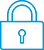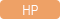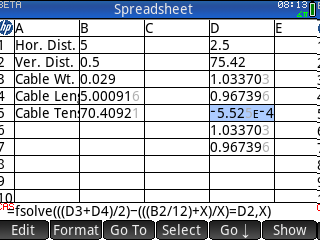cancel
Showing results for
Search instead for
Did you mean:It has been a while since anyone has replied. Simply ask a new question if you would like to start the discussion again.Level 2
12 10 1 1
Message 1 of 16
1,624
Flag Post

Solved!

# How to use the HP Prime Solve app in the Spreadsheet app? Ref: Excel add in 'Solver'HP Recommended
HP Prime Calculator

MS Excel has an add in called 'Solver'.  In it you are asked for an objective cell, a value to shoot for (like 0.000001), and another cell called 'By changing variable cell:'.  It also asks for a solving method such as 'GRG Nonlinear'.  My question is:  Does the HP Prime spreadsheet have anything like this?  Maybe using the Prime's Solver app within its spreadsheet?  If so, could you please tell me how to use it?  I am trying to write  a spreadsheet to calculate the tension in a catenary cable which requires iterating a value using a computer type solver.  Thank you for any help.  -  Jimpilot1

Tags (1)
15 REPLIES 15Level 6
252 251 42 77
Message 2 of 16
Flag PostHP Recommended

Hello,

Use the CAS fsolve function.

For example, I set, A1 as =fsolve(x²-2*x-1=B1,x) and a numerical value in B1.

Note, since this A1 is a CAS formula, when you enter it in the spreadsheet, you need to click on the "cas" menu item BEFORE pressing the enter key, else you will get an error.

With Prime, no need for addins! it's build in...

Cyrille

I am an HP EmployeeLevel 2
12 10 1 1
Message 3 of 16
Flag PostHP Recommended

Thank you, Cyrille.  I tried the fsolve function and it seemed to work.  Actually it did work as soon as I entered the CAS formula.  That is, I got the right answer for the input data I had entered.  However, when I entered different input data, the answer remained the same as for the old input data.  Is there a way to force a recalculation for the spreadsheet?  Again, thank you very much for your help.  -  JimLevel 6
252 251 42 77
Message 4 of 16
Flag PostHP Recommended

hello,

What formula did you use?

You got an answer as soon as you entered the formula because an empty cell returns 0...

But normally, all the cell recalculate when you change a cell, so it should have changed, unless the result for the different value you entered is the same (or the first characters look the same) as the result for 0.

Cyrille

I am an HP EmployeeLevel 2
12 10 1 1
Message 5 of 16
Flag PostHP Recommended

Hi Cyrille -  The formula is:  =fsolve((D3+D4)/2-(B2/12+X)/X=D2,X)   The formula is in cell D5 and shows {} which I guess is the calculator's way of saying that the formula will be calculated in CAS.  The iteration of the formula should drive the value towards zero while driving the value in cell D2 towards a value used to solve the catenary problem.  If you would like, I would be happy to send you the complete spreadsheet.  It only consists of 3 columns and 7rows.  -  JimLevel 2
12 10 1 1
Message 6 of 16
Flag PostHP Recommended

Hi again - To save time I'll show my complete spreadsheet here:

Catenary Cable:

A1:  Hor. Dist.  (Ft)                B1:  5.00                                           D1:  =B1/2

A2:  Ver. Dist.  (In)                 B2:  0.5                                              D2:  75.42  (Number generated by fsolve function)

A3:  Cable Wt.  (lbs/Ft)         B3:  0.029                                         D3:  =EXP(D1/D2)

A4:  Cable Length  (Ft.)        B4:  =(D6-D7)*D2                            D4:  =1/D3

A5:  Cable Tension  (lbs)      B5:  =( B2/12+D2)*B3*32.174     D5:  =fsolve((D3+D4)/2-(B2/12+X)/X=D2,X)

D6:  =EXP(D1/D2)

D7:  =1/D6

Cells B1, B2, and B3 are the input data cells.  Cells B4 and B5 are the output data cells.  I hope that the spacing

and format won't be messed up when I post this!  Thank you so much, Cyrille, for helping me.  -  JimLevel 6
252 251 42 77
Message 7 of 16
Flag PostHP Recommended

Hello,

I think that you are mis-understanding the fsolve line...

it is trying to find X so that the expression (D3+D4)... is equal to D2. It will NOT change the value of D2...

Here is what I have, and the value displayed in D5 changes when I change B1, B2 or B3...I am not understanding the working of your system... Is this an iterative thing where you take the previous result of the fsolve and copy it to D2 and restart?

In this case, you would need to change the formula in D5 to

=D2:=fsolve(((D3+D4)/2)-(((B2/12)+X)/X)=D2,X)

so that the result of the fsolve does get stored in D2... But then you need to force the spreadsheet to recalculate the whole sheet again and again...

The spreadsheet will recalculate anytime somethign is changed by the user (the value of a cell).. Do this by manually entering a value in any cell repetitively..

Alternatively, you can force the spreadsheet to recalculate every time it displays (on every key or touch press)... To do this, turn caching OFF. This is an option in the "format" menu of the cell 0,0 (the one with the HP logo).

I did do these changes, but the spreadsheet does not seem to converge toward a solution (it does converge, but the result is not a solution to the problem as far as I can tell).. maybe something to debug... Good luck! and I hope that this helped!

Cyrille

I am an HP EmployeeLevel 2
12 10 1 1
Message 8 of 16
Flag PostHP Recommended

Hi Cyrille - It seems that we are getting closer.  I changed your D5 formula to:

=D2:=fsolve(((D3+D4)/2)-(((B2/12+D2)/D2=.000001,D2)     I turned caching NO and can now force the spreadsheet to recalculate.  However, with each iteration the value in cell D5 just grows.  It should be going towards zero - or at least towards 0.000001.  I'll post another Catenary spreadsheet than I have written in which the user guess values for cell D2.  If you play with this 'manual' spreadsheet you will see what I am trying to do with fsolve.   (Automate the spreadsheet so that the user will not have to input guesses.)  Thanks for your help! - JimLevel 2
12 10 1 1
Message 9 of 16
Flag PostHP Recommended

Hi again -  Here is my 'manual' Catenary Cable' spreadsheet:

Catenary Cable:

A1: Hor. Dist. (Ft)                             B1: 5.00                                                        D1:   =B1/2

A2: Ver. Dist. (In)                              B2: 0.5                                                           D2:   =EXP(D1/B4)

A3: Cable Wt. (lbs/Ft)                     B3: 0.029                                                       D3:   =1/D2

A4:  GUESS >                                    B4:  75.01                                                      D4:   =EXP(D1/B4)

A5:  Make 0 By Guessing >           B5:  =(D2+ D3)/2-(B2/12+B4)/B4           D5:   =1/D4

A6: Cable Length (Ft.)                    B6: =(D4-D5)*B4

A7: Cable Tension (lbs)                  B7: =( B2/12+B4)*B3*32.174

Cells B1, B2, and B3 are the input data cells. Cell B4 is any guess within reason.   Cell B5 goes towards 0 the nearer cell B4 gets to cell B5 solution.  User narrows down the guesses by looking at the sign (+/-) in cell B5.    Cells B6 and B7 are the output data cells.  I fill (format) cell B5 with yellow so as to not accidentally  put my guesses in a wrong cell.

I use this spreadsheet when designing control cables runs for my homebuilt experimental airplanes.  My calculations would go a lot faster if we can automate the guess work.   Thank you again, Cyrille, for your help. --  JimLevel 6
252 251 42 77
Message 10 of 16
Flag PostHP Recommended

Hello,

You can of course automate the problem by writing a program, not a spreadsheet sheet...

Cyrille

I am an HP Employee
† The opinions expressed above are the personal opinions of the authors, not of HP. By using this site, you accept the Terms of Use and Rules of Participation